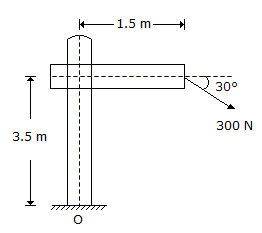# Civil Engineering - UPSC Civil Service Exam Questions

1.

Tensile strength of concrete is measured by

 A. direct tension test in the universal testing machine B. applying compressive load along the diameter of the cylinder C. applying third point loading on a prism D. applying tensile load along the diameter of the cylinder

Explanation:

No answer description available for this question. Let us discuss.

2.

What is the moment (approximate) of the force acting on a railway sign post shown in the figure about the point ?A. 1135 Nm B. 1205 Nm C. 980 Nm D. 1300 Nm

Explanation:

No answer description available for this question. Let us discuss.

3.

A flash mixer of 2.0 m3, with a velocity gradient of mixing mechanisms equal to 660/s, and fluid absolute viscosity of 1.0 x 10-3 Ns/m2 is continuously operated. What is the power input per unit volume ?

 A. 360 W B. 720 W C. 1440 W D. 300 W

Explanation:

No answer description available for this question. Let us discuss.

4.

What is the discharge corresponding to a critical depth of 1.20 m in a 3.0 m wide rectangular channel:

 A. 4.12 m3/s B. 4.94 m3/s C. 8.24 m3/s D. 12.35 m3/s

Explanation:

No answer description available for this question. Let us discuss.

5.

A lemniscate curve between the tangents is transitional throughout, if the polar deflection angle of its apex is equal to (φ in the deflection angle between the initial and final tangents)

 A. φ/2 B. φ/4 C. φ/6 D. φ